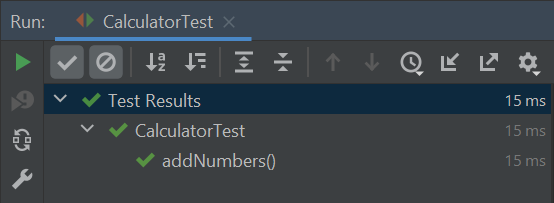Getting Started with JUnit 5: Writing Your First TestIn this article, we will learn how to write and run a simple JUnit 5 test. We will learn how to setup preconditions, interact with the object we want to test, and verify that it behaves like we expect.

Setup

The accompanying source code example already has configurations for both Maven and Gradle but there are also step by step guides for our convenience:

Writing Our First Test

When we are testing some piece of software we want to make sure it does what it is expected to do. When writing automated tests:

1. First, we set up preconditions for code we want to test
2. Next, we interact with the code to test
3. Finally, we check the results are what we expected them to be

This is called the Arrange, Act, Assert pattern.

Let’s take a look at some piece of software that we would like to test:

public class Calculator {
public int add(int first, int second) {
return first + second;
}
}

We have a very simple calculator class. All it does is add two numbers together. When testing this code, we would like to make sure the calculation is correct.

We can follow the Arrange, Act, Assert pattern by:

• First constructing an instance of the Calculator class
• Then calling the add() method with some parameters and store the result
• Finally, checking the results by calling the assertEquals() method that compares the expected and actual value
import org.junit.jupiter.api.Assertions;
import org.junit.jupiter.api.Test;

class CalculatorTest {
@Test
// Arrange
Calculator calculator = new Calculator(); <strong>x</strong>

// Act

// Asset
Assertions.assertEquals(3, sum);
}
}

The test class can be called anything but here we have put the test code in a CalculatorTest class. We will name the test method simply addNumbers() so that it describes what we are trying to do.

To be able to run the test, we also have to annotate the method with the @Test annotation. This way the test runner recognizes the method as a test method.

Running Our Test

To run the tests we have several options. When using an IDE we can run the tests directly from the IDE. We can also run the test from the command line using a build tool like Maven or Gradle.

When the software performs as expected, the test will pass. When it does something unexpected, the test will fail.

Running from IntelliJ IDEA

When using an IDE like IntelliJ IDEA we can just right click the test class and select Run CalculatorTest. Alternatively we can just use the Ctrl+Shift+F10 (Windows) or Ctrl+Shift+R (Mac) shortcut to run the tests.

Here we can see the results of running a passing JUnit 5 test in IntelliJ IDEA:Running with Maven

To run the tests from the command line using Maven:

\$ mvn test

We should see output similar to this:

[INFO] -------------------------------------------------------
[INFO]  T E S T S
[INFO] -------------------------------------------------------
[INFO] Running com.arhohuttunen.CalculatorTest
[INFO] Tests run: 1, Failures: 0, Errors: 0, Skipped: 0, Time elapsed: 0.021 s - in com.arhohuttunen.CalculatorTest
[INFO]
[INFO] Results:
[INFO]
[INFO] Tests run: 1, Failures: 0, Errors: 0, Skipped: 0
[INFO]
[INFO] ------------------------------------------------------------------------
[INFO] BUILD SUCCESS
[INFO] ------------------------------------------------------------------------

To run the tests from the command line using Gradle:

We should see output similar to this: